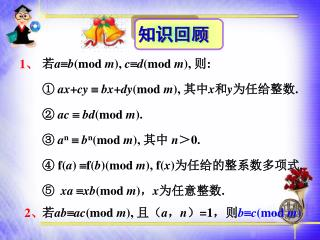# 知识回顾 - PowerPoint PPT PresentationDownload PresentationDownload Presentation## 知识回顾

- - - - - - - - - - - - - - - - - - - - - - - - - - - E N D - - - - - - - - - - - - - - - - - - - - - - - - - - -
##### Presentation Transcript

1. 知识回顾 若ab(mod m), cd(mod m), 则: ① ax+cybx+dy(mod m), 其中x和y为任给整数. ② acbd(mod m). ③ anbn(mod m), 其中 n＞0. ④ f(a) f(b)(mod m), f(x)为任给的整系数多项式. ⑤ xaxb(mod m)，x为任意整数. 1、 若abac(mod m), 且（a，n）=1，则bc(mod m) 2、

2. 导入新课 我们以前已经学过了验证运算结果的正确性，对于加法我们用减法来检验，乘法我们用除法来检验.如：25-3=22，正确与否我们用加法验证，因为：22+3=25，所以结果正确.对于很大的数值运用此方法就有局限性了.

3. 对于运算量很大的式子，怎样很快的判断其正确性呢？如：1234567×147895=456789415对于运算量很大的式子，怎样很快的判断其正确性呢？如：1234567×147895=456789415 你能很快的判断其正确行吗？

4. 当数目很大时，对运算结果正确性的判断就变的比较麻烦了. 如：1234567×147895=456789415 5789461-159634=4789657 78561238+75894=123456752等. 要想很快的判断其正确性，就要学一种新的方法——弃九验算法.

5. 第二讲 同余与同余方程 第六节 弃九验算法

6. 教学目标 知识与能力 1、会用弃九验算法验算正整数的计算结果是否正确.2、知道弃九验算法只能“检错”，不能“判正”.

7. 过程与方法 1、通过以前知识的引用，循序渐进的推导出弃九验算法.2、通过实例解析对所学的知识进行巩固. 情感态度与价值观 培养学生勇于探究、研究的精神和兴趣.能够举一反三将所学的知识应用的减法、加法、除法中.

8. 教学重难点 重点 难点 用弃九验算法验算正整数的计算结果是否正确.知道弃九验算法只能“检错”，不能“判正”. 弃九验算法的推导过程.

9. 我们知道一个正整数可以写成各个位数与十的幂的乘积的和的形式. 如：35=3×10+5； 789=7×102+8×10+9 4236=4×103+2×102+3×10+6对于任意的自然数 应该怎样表示呢？ 探究

10. 按以上的形式类推可表示成 N=an×10n+…+a2×102+a1×10+a0 =an×（99…9+1）+…+a2×（99+1）+a1（99+1）+a0 =9（11…1 an +..+11 a2 + a1）+（a0+a1+…+an） 可以看出9︱N- （a0+a1+…+an） ， 所以N≡ a0+a1+…+an（mod9） 我们得到这个整数和它的各位数字之和模9同余.

11. 设有整数x1，x2，x3，它们的各位数字之和分别为x1’，x2 ’，x3 ’由上面的推论我们可以得到x1≡ x1’（mod9），x2≡ x2’（mod9）， x3≡ x3’（mod9）， 由同余的性质若ab(mod m), cd(mod m), 则ac bd(mod m). 得到x1 x2 ≡ x1’ x2’（mod9），若x3=x1x2，则 x1’ x2’ ≡ x3’（mod9），

12. 1、判断2164×7895=45680的正确与否. 解析2164≡2+1+6+4=13≡4（mod9） 7896≡7+8+9+6=30≡3（mod9） 45680≡4+5+6+8+0=23≡5（mod9） 因为 所以式子不正确. 实例 学以致用吆！

13. 拓展一 已经学会了在乘法中运用弃九验算法，由于乘法和除法是互逆，我们将弃九验算法应用到除法当中.如：A÷B=C,我们可转化成B×C=A,来用弃九验算法.

14. 2、判断12345÷47=4567的正确与否. 解析：由乘法与除法互为逆运算， 则47×4567=12345， 于是47≡4+7=11≡2（mod9） 4567≡4+5+6+7=22≡4（mod9） 12345≡1+2+3+4+5=15≡6（mod9） 由于故式子不正确. 应用

15. 拓展二 对于加法、减法结合同余性质 若： ab(mod m), cd(mod m), 则: a+cb+d(mod m), 一样可以运用弃九验算法，由于加法和减法是互逆，我们只要学会了加法的弃九运算，就会应用到减法中了.

16. 3、判断45318+4518=45416的正确与否. 解析： 45318≡4+5+3+1+8=21≡3（mod9） 4518≡4+5+1+8=18≡0（mod9） 45416≡4+5+4+1+6=20≡2（mod9） 由于故式子不正确. 应用

17. 课堂小结 一、弃九验算法则 ： 若做运算的正整数的各位数字之和模9的余数做相应运算不等于运算结果的正整数的各位数字之和，模9的余数则一定不正确.

18. 二、弃九验算局限性：只能验证错误，不能验证正确. 三、弃九验算应用：可以用来快速的判断乘法、除法、加法、减法运算结果的正确与否.切记只能判错，不能判对.

19. 高考链接 1、将自然数1，2，3，…依次无间隔地写下去组成一个数1234567891011213…如果一直写到自然数100，所得的数除以9的余数是多少？ 分析与解：因为这个数太大，在使用弃九法时不能逐个划掉和为9或9的倍数的数，

20. 因为1＋2＋3＋…＋9＝45，而45是9的倍数，所以每一组1，2，3，…，9都可以划掉. 而1～99这九十九个数中，个位数有十组1，2，3，…，9，都可划掉；十位数也有十组1，2，3，…，9，也都划掉. 最后只剩下的100中的数字1. 所以这个数除以9余1.

21. 解析：利用弃九验算法 46876≡4+6+8+7+6=31≡4（mod9） 9537≡9+5+3+7 =24≡6（mod9） 4476652≡4+4+7+6+6+5+2=34≡7（mod9） 由于 所以 式子不成立了 2、检验下面的乘法算式是否正确： 46876×9537＝4476652.

22. 解析：利用弃九验算法 只需验证123 × 46=5558 123≡1+2+3=3≡6（mod9） 46≡4+6 =9≡0（mod9） 5558≡5+5+5+8=23≡5（mod9） 由于 所以 123 × 46=5558式子不成立即原式不成立. 3、验证是否正确 5558÷ 46= 123

23. 课堂练习 1、多位数521983除以9的余数是（ ）. 2、多位数215938343与多位数593867的余数 是否相同（ ）. 1 相同

24. 3、多位数2638457除以9的余数是（ ）. A.7 B.8 C.9 D.5 B 4、多位数12907225除以9的余数是（ ）. A.1 B.4 C.2 D.7 A

25. 5、求多位数7645821除以9的余数. 解析：利用弃九验算法 由于 7645821≡7+6+4+5+8+2+1=33 ≡6（mod9） 所以 7645821除以9的余数是6

26. 解析：利用弃九验算法 7832145≡7+9+3+2+1+4+5=31≡4（mod9） 2167953≡2+1+6+7+9+5+3=33≡6（mod9） 5664192≡5+6+6+4+1+9+2=33≡6（mod9） 由于 所以 式子不成立 6、检验下面的减法算式是否正确：　　 7832145-2167953＝5664192.

27. 解析：利用弃九验算法 2638457≡2+6+3+8+4+5+7≡8（mod9） 3521983≡3+5+2+1+9+8+3≡4（mod9） 6745785≡6+7+4+5+7+8+5≡6（mod9） 12907225≡1+2+9+0+7+2+2+5≡1（mod9） 由于所以 式子不成立. 7、检验下面的加法算式是否正确： 2638457＋3521983＋6745785＝12907225.

28. 教材习题答案 1、1524≡1+5+2+4=12 ≡3（mod9）， 3456≡3+4+5+6=18 ≡0（mod9）， 4880≡4+8+8+0=20 ≡2（mod9）， 由于 故算术不正确. 习题(第32页) 2、只需验算式2346+1340=2596是否正确. 2346≡2+3+4+6=15 ≡6（mod9），

29. 1340≡1+3+4+0=8 ≡8（mod9）， 2596≡2+5+9+6=22 ≡4（mod9）， 由于 故算式不正确. 3、4328≡4+3+2+8=17 ≡8（mod9）， 3249≡3+2+4+9=18 ≡0（mod9）， 14246432≡1+4+2+4+6+4+3+2=26 ≡8（mod9）， 由于 故算式不正确.

30. 4、只需验算算式 165×1432=226380是否正确. 165≡1+6+5=12≡3（mod9）， 1430≡1+4+3+2=10≡1（mod9）， 226380≡2+2+6+3+8+0=21≡3（mod9），而3×1≡3（mod9）,故用弃九验算法无法判断算式是否正确.事实上,直接计算发现,该算式是错误的.